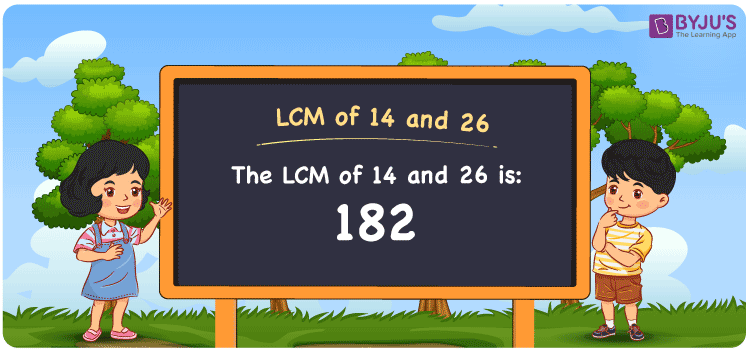# LCM of 14 and 26

LCM of 14 and 26 is 182. The Least Common Multiple or Lowest Common Multiple always known as LCM is the smallest positive numeral that is divisible by the given group of numbers. In the given set of numbers 14 and 26, 182 is the first (least or smallest) number that is common in the set of multiples of 14 and 26. The LCM is also known as LCD, Least Common Divisor. You can use the LCM with Examples to learn more about lcm.

## What is LCM of 14 and 26

The Least Common Multiple or Lowest Common Multiple of 14 and 26 is 182.## How to Find LCM of 14 and 26?

LCM of 14 and 26 can be determined using three methods:

• Prime Factorisation
• Division method
• Listing the multiples

### LCM of 14 and 26 Using Prime Factorisation Method

In the Prime Factorisation method, the numbers 14 and 26 can be expressed as;

14 = 2 × 7

26 = 2 × 13

2 is a common factor for both 14 and 26, and other factors 7 and 13 are prime, the product of these three numbers forms the LCM.

LCM (14, 26) = 2 × 7 × 13 = 182

### LCM of 14 and 26 Using Division Method

In the Division Method, the numbers 14 and 26 are divided together. The product of common and uncommon prime divisors of 14 and 26 forms the LCM.

 2 14 26 7 7 13 13 1 13 × 1 1

Hence, LCM (14, 26) = 2 × 7 × 13 = 182

### LCM of 14 and 26 Using Listing the Multiples

By listing all the multiples of 14 and 26, we can identify the LCM. Below is the list of multiples for 14 and 26

Multiples of 14:

14, 28, 42, 56, 70, 84, 98, 112, 126, 140, 154, 168, 182, 196, 210….

Multiples of 26:

26, 52, 78, 104, 130, 156, 182, 208, 234,….

LCM (14, 26) = 182

## Video Lesson on Applications of LCM## Solved Examples

What is the smallest number that is divisible by both 14 and 26?

Answer: 182 is the smallest number that is divisible by both 14 and 26.

What is the LCM for 13, 14 and 26?

Answer: LCM for 13, 14 and 26 is 182.

## Frequently Asked Questions on LCM of 14 and 26

### What is the LCM of 14 and 26?

The LCM of 14 and 26 is 182.

### Is the LCM of 14 and 26 the same as the Highest Common Factor of 14 and 26?

No. LCM of 14 and 26 is 182 and Highest Common Factor of 14 and 26 is 2.

### What is common in the numbers 14 and 26?

1 and 2 are common in the numbers 14 and 26.

### What are the first 3 common multiples of 14 and 26?

The first three common multiples of 14 and 26 are 182, 364, and 546.

### Is the LCM of 7 and 14 same as the LCM of 13 and 26?

No. LCM of 7 and 14 is 14, whereas the LCM of 13 and 26 is 26. They are not the same.